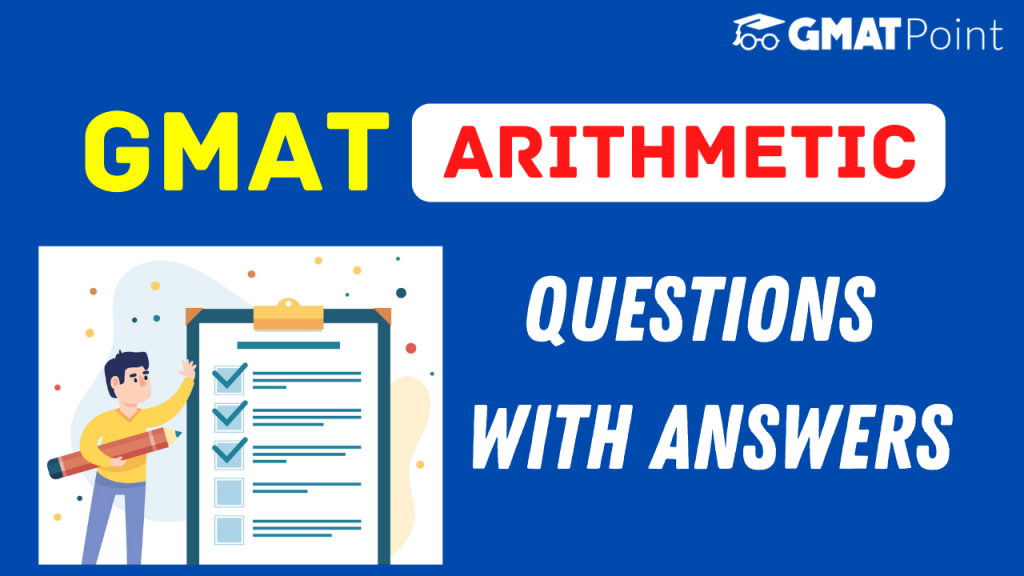# GMAT Arithmetic Questions With Answers# GMAT Arithmetic Questions With Answers

Arithmetic questions constitute a major portion of the Quant section of the GMAT. These questions can come under both Problem Solving and Data Sufficiency types. Arithmetic has a number of topics in it. In this article, we will be looking into –

1. GMAT Arithmetic Topics
2. GMAT Arithmetic Questions with Answers

## GMAT Arithmetic Topics

In the GMAT, Arithmetic constitutes a number of topics. They are as follows(this list is in accordance to the GMAT Official Guide):

1. Integers, Fractions, Decimals – Numbers
2. Ratios and Percentages
3. Powers and Roots of Numbers
4. Statistics
5. Set Theory
6. Counting and Probability

## Examples – GMAT Arithmetic Questions

### Question 1:

If a regular unbiased die is rolled twice, what is the probability of getting a sum greater than 10 in the two rolls?

### Approach:

In probability, the probability of an event E = The number of favourable outcomes / Total number of outcomes

The first step is to calculate all favourable outcomes.

Let {x,y} represent the two rolls.

For getting a sum greater than 10, we can get a sum of 11 it a sum of 12.

Now, for a sum of 11, we can have these cases – {5,6}, {6,5}

For a sum of 12, we can have these cases – {6,6}

Hence, there are three favourable outcomes.

Total number of outcomes = 6*6 = 36

Hence, probability = 3/36 = 1/12.

### Question 2:

If the ratio of A:B = 2:3 and the ratio of B:C is 2:3, find out the ratio of the sum of A and C to the absolute difference of A and C.

### Approach:

A:B = 2:3

B:C = 2:3

SInce B is common, we take the LCM of (2,3) = 6

Now, in the final ratio, A:B = 4:6

B:C = 6:9

A:B:C = 4:6:9

A + C = 13

|A – C| = 5

Ratio = 13:5

### Question 3:

If a set A has 16 distinct elements all of which are integers and set B necessarily has the square of all elements of set A, what can be the minimum number of elements in set B?

### Approach:

If all 16 elements in A are distinct, say 1, 2, 3, …., 16, then B also has 16 elements 1, 4, 9, ….., 256.

However, to reduce the number of elements of B, we need to have as many elements as possible in A that also has their negative counterpart in A. For example, if 2 is an element, -2 should be an element as well. As a result, if 4 (square of both 2 and -2) is present in B, it will account for 2 elements in A.

Hence, we can have 8 positive numbers in A and their corresponding negative numbers in A. In this way, we can say that B will have a minimum of 8 elements.

Hence, the minimum possible number of elements in B is 8.

### Note:

Though these examples provide a good sense of what type of GMAT ARithmetic questions you can expect, in no way do they represent the exhaustive list of concepts required for the Quantitative section of the GMAT.

### Tips to keep in mind:

1. Try to take some time out from the Problem-Solving Arithmetic questions so that you can use that time to solve the tricky Data Sufficiency Arithmetic questions.
2. Do not get stuck in a question for long. If you find yourself trapped in a question for long, take a guess and move on.